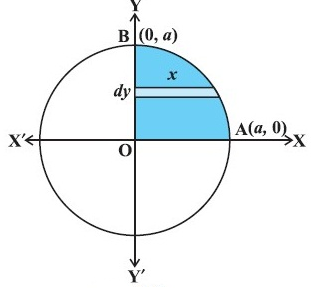### Common Errors in Secondary Mathematics

Common Errors Committed  by the  Students  in Secondary Mathematics   Errors  that students often make in doing secondary mathematics  during their practice and during the examinations  and their remedial measures are well explained here stp by step.  Some Common Errors in Mathematics

### Lesson Plan Math Class 12 (Ch-8) | Application of Integral

E- LESSON PLAN   SUBJECT MATHEMATICS    CLASS 10+2
Lesson Plan Class 12th Subject Mathematics for Mathematics Teacher. Effective way of Teaching Mathematics. Top planning by the teacher for effective teaching in the class.
 Board – CBSE CLASS –XII SUBJECT- MATHEMATICS CHAPTER 8  :- Applications of  Integrals

TOPIC:- Chapter : 8 : Applications of Integrals

DURATION:-

This chapter is divided into 4 modules and is completed in 10 class meetings.

PRE- REQUISITE KNOWLEDGE:-

Knowledge of trigonometry Chapter 3 class 10+1,
inverse trigonometry Chapter 2 class 10+2,
Indefinite Integration chapter 7 class 10+2
Definite Integral Chapter 7 Class 10+2

TEACHING AIDS:-

Green Board, Chalk,  Duster, Charts, smart board, projector, laptop etc.

METHODOLOGY:-   Lecture method

OBJECTIVES:-

Ø Applications in finding the area under simple curves, especially lines, circles, parabolas, ellipses (in standard form only).
Ø The area between any of the two above said curves (the region should be clearly identified).

PROCEDURE :-

Start the session by asking the questions related to the trigonometry, inverse trigonometry and formulas of Integration. Now introduce the topic application of Integral step by step as follows.

 S.N Topic 1 IntroductionFirst of all teacher should recapitulate the concept of drawing the graphs of straight line and other curves like Circle, Parabola, Ellipse and Hyperbola and make the students aware with their general equations. 2 Now teacher will introduce the topic to find the area under the simple curve like lines and circles, parabola and hyperbola. 3 Teacher should explain the concept of finding the area between two curves in combinations. 4 Teacher can provide some more problems to explore the knowledge of the studentEXPECTED OUTCOMES:-

After studying this lesson students should know
• the method drawing the graphs of line, circle, parabola, ellipse and hyperbola.
• students should knows the method of finding the area between any two above said curves.
STUDENTS DELIVERABLES:-
• Review questions given by the teacher.
• Students should prepare the presentation in groups on the method of finding the area between any of the two curves.
• Solve NCERT problems with examples.
EXTENDED LEARNING:-

Students can extend their learning in Mathematics through the RESOURCE CENTRE.  Students can also find many interesting topics on mathematics at cbsemathematics.com

ASSESSMENT TECHNIQUES:-
• Assignment sheet will be given as home work at the end of the topic.
• Separate sheets which will include questions of logical thinking and Higher order thinking skills will be given to the above average students.
• Class Test , Oral Test , worksheet and Assignments. can be made the part of assessment.
• Re-test(s) will be conducted on the basis of the performance of the students in the test.

🙏